# Draw Dot Plot Using Python and Matplotlib

Matplotlib doesn't support dot plots natively. So how can you draw them?

Let's write our own function and use it to sketch highly customizable dot plots.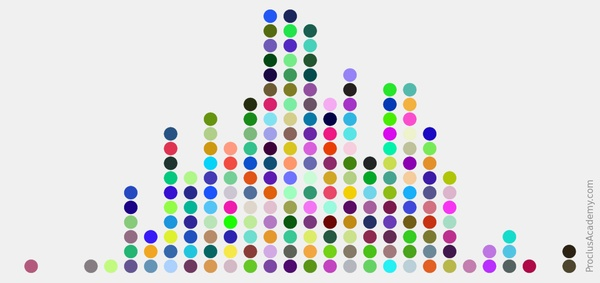# Precision, Recall, and F1 Score: A Practical Guide Using Scikit-Learn

How can we use Scikit-Learn to measure Precision, Recall, and F1 Score for classification models?

Also, does Scikit-Learn provide a way to handle imbalanced classes?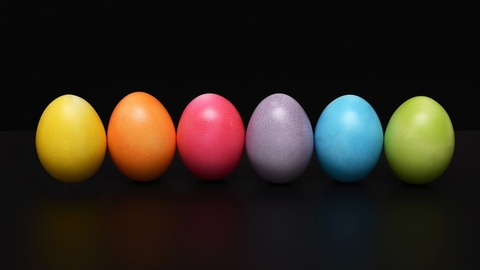# Precision, Recall, and F1 Score: When Accuracy Betrays You

Accuracy can be a misleading metric for certain types of classification problems.

Let's explore how Precision, Recall, and F1 Score can give a realistic view of a model’s predictive power.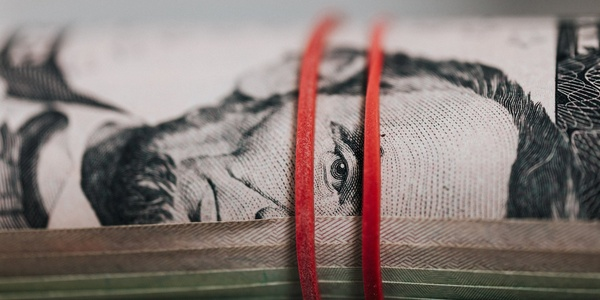# 3 Regression Metrics You Must Know: MAE, MSE, and RMSE

This post demystifies the most common metrics used to evaluate regression models.

You'll also gain practical skills to generate these metrics using Scikit-Learn.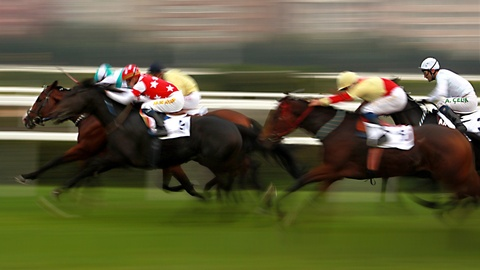# Normal Distribution and the Empirical Rule

This post introduces you to Normal Distribution and some of its distinctive features.

You'll also learn about Empirical Rule, which dictates how values are spread in specific intervals around the mean.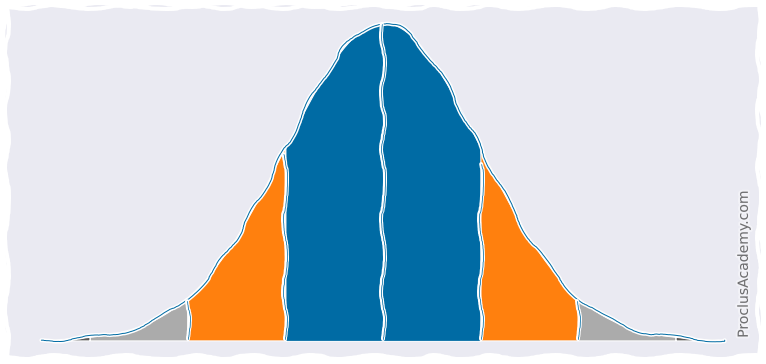# Area Under Density Curve: How to Visualize and Calculate Using Python

Let's explore Area Under Density Curve. What does it represent? What are some of its practical applications?

You'll also learn to plot and analyze partial areas under the curve using Matplotlib, Seaborn, and Numpy.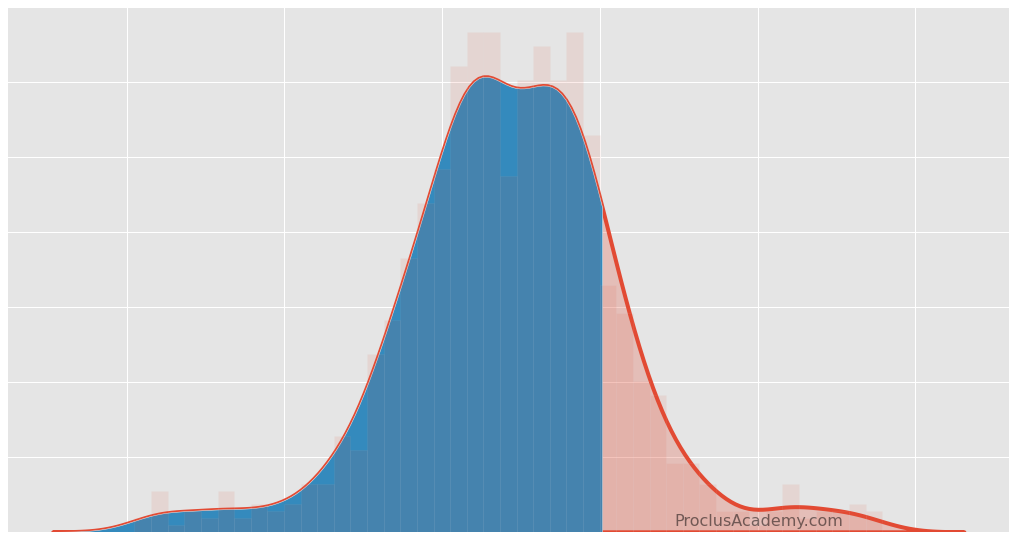# Data Distribution, Histogram, and Density Curve: A Practical Guide

Let's explore how Data Distribution enables you to extract general patterns from the data.

You'll also learn to visualize distribution as Histogram and Density Curve using Matplotlib and Seaborn.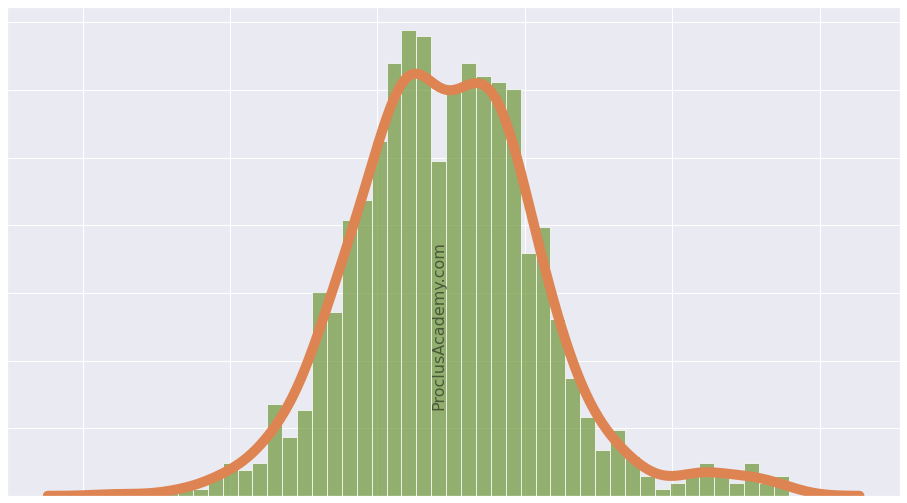# How to Customize Pie Charts using Matplotlib

Let's explore how you can use Matplotlib to draw pie charts with customized colors and labels. You can even apply styles tailored to each slice.

Along the way, you'll see what's an exploding pie chart and how to draw it. Finally, you'll learn to plot Donut Charts!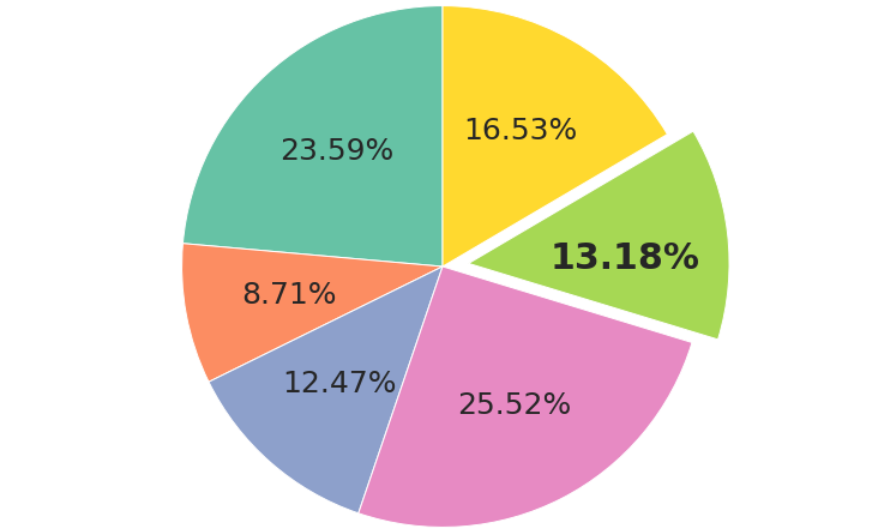# What Is Stratified Sampling and How to Do It Using Pandas?

Do you want samples that accurately represent the population? Here's how Stratified Sampling can help.

You'll also develop practical skills and learn how to do sampling using Python and Pandas.# How to Generate Datasets Using make_classification

Let's explore how to create classification datasets with balanced or imbalanced classes and binary or multiclass labels.

You can even produce datasets that are harder to classify!